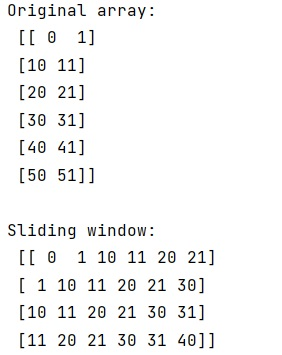# Sliding window of MxN shape numpy.ndarray()

Learn, how to get a sliding window of MxN shape from a numpy.ndarray?
Submitted by Pranit Sharma, on February 14, 2023

NumPy is an abbreviated form of Numerical Python. It is used for different types of scientific operations in python. Numpy is a vast library in python which is used for almost every kind of scientific or mathematical operation. It is itself an array which is a collection of various methods and functions for processing the arrays.

## Getting a sliding window of MxN shape from a numpy.ndarray

Suppose that we are given a numpy array of some arbitrary shape (say 6,2), and we need a sliding window with step size 1 and window size (let us say 3).

For this purpose, we can do a vectorized sliding window in numpy using fancy indexing. We will first create a numpy array and then we will work on creating a window. To create a window, we will slice the numpy array using None.

Let us understand with the help of an example,

## Python code to get a sliding window of MxN shape from a numpy.ndarray

```# Import numpy
import numpy as np

# Creating a numpy array
arr = np.array([[00,1], [10,11], [20,21], [30,31], [40,41], [50,51]])

# Display original array
print("Original array:\n",arr,"\n")

# Flattening the array
fl = arr.flatten()

# Getting the indices of sliding window
ind = np.arange(6)[None, :] + np.arange(4)[:, None]

# Getting the values with indices
res = fl[ind]

# Display sliding window
print("Sliding window:\n",res)
```

Output: